# Left-Handed Neutrinos

The relative orientations of spin and linear momentum for neutrinos and antineutrinos is apparently fixed and intrinsic to the particles.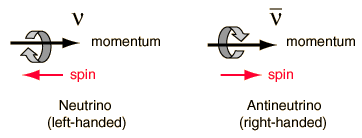This property is related to the concept of parity and to the conservation laws which apply to particle interactions.

For neutrinos the spin is always opposite the linear momentum and this is referred to as "left-handed", whereas the antineutrinos are always "right-handed". This evokes the picture of the right-hand rule for vector angular momentum. In this convention, the fingers of the right hand are used to indicate the sense of the spin or orbital motion, with the thumb pointing in the direction of the defined angular momentum. The momentum of the particle is used to define a preferred direction in space, and if you curled the fingers of your right hand to show the sense of the "spin" of the antineutrino, your thumb would point in this momentum direction. Hence it is a "right-handed" particle. For the neutrino, you would have to use the fingers of your left hand to get your thumb to point in the direction of the linear momentum.

Prior to 1956 all the available experimental evidence suggested that "left-handed" vs "right-handed" was not a meaningful characterization of particle interactions since the laws of physics appeared to conserve parity. Interactions which proceed by the strong and electromagnetic were shown by experiment to "conserve parity". But the theoretical work of Lee and Yang and the experiment with cobalt-60 decay by Wu showed that this decay by the weak interaction did not conserve parity. This causes the weak interactions which emit neutrinos or antineutrinos to violate conservation of parity.

The property which has been called left-handed and right-handed here is called "helicity". The helicity of a particle is defined as the ratio ms/s, or the z-component of spin divided by the magnitude of the spin. By this definition in this case, the helicity is +1 for a right-handed antineutrino and -1 for a left-handed neutrino.

 Neutrinos as leptons Role in supernova Other neutrino types
Index

Reference
Griffiths, 2nd Ed
Sec 4.4

 HyperPhysics***** Quantum Physics R Nave
Go Back

# Neutrino Cross Section

 The nominal neutrino cross section for interaction with a nucleon increases with energy as indicated by the data at right cited by Rohlf. The data displayed at right is taken at energies far above the range of neutrinos from radioactive decay. The slope of the straight line at right, when extrapolated down to 1 MeV, gives a cross section more like 10-45 m2. This is still three orders of magnitude larger than the cross-section measured by Cowan & Reines in their pioneering experiment. Rohlf's comment was that this linear energy dependence of the cross-section was for neutrino energies large compared to the mass energies of the quarks, so an extrapolation to the energies of ordinary radioactive decay is asking too much.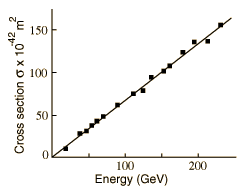The original neutrino detection by Reines and Cowan was based on the reaction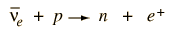This interaction is related by crossing symmetry to the decay of the neutron, the simplest example of beta decay. It is in fact sometimes referred to as "inverse beta decay". So it would appear that the study of beta decay could give an approach to the cross-section for the neutrino interaction.The approach to calculating the cross-section for the interaction is based on Fermi's Golden Rule , and if a matrix element can be found for the weak interaction in one of these reactions it should be comparable in the other.

Estimates of the weak interaction coupling strength come from applying the Fermi theory of beta decay in some special cases. Krane does this to arrive at an estimate of 10-43 cm2 = 10-47 m2 for the neutrino cross section in the beta decay range of energy. This is about 20 orders of magnitude less than the scattering cross-section for two nucleons at low energy!

With this nominal cross section, some estimates of rates of interaction can be made. Multiplying the cross section times the nucleon density gives a number of interactions per meter, and the inverse of that is an estimate of the mean free path. For water with a density of 1000 kg/m3, the mean free path for a neutrino can be estimated fromOne perspective on these distances is to compare to a light year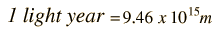So this estimate of mean free path is more than a light year of lead! A fairly common qualitative statement in physics texts is that the mean free path of a neutrino is about a light-year of lead. Griffiths makes the statement "a neutrino of moderate energy could easily penetrate a thousand light-years(!) of lead." This cross section can also be used to estimate the number of events which can be expected in a given size of detector.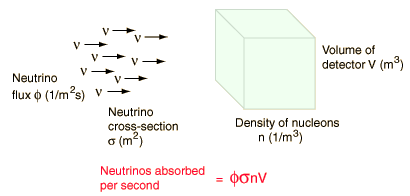The Bahcall calculation of the solar neutrino flux is about 5 x 106 /cm2s at the Earth's surface. In what is called the "solar neutrino problem", only about a third to one half this many were measured in early experiments. Current experiments at the Sudbury Neutrino Observatory suggest that neutrino oscillation transmutes some of the solar electron neutrinos into muon and tau neutrinos. When that is taken into account in the recent SNO experiments, the flux is in close agreement with the Bahcall estimate.

 Neutrinos as leptons Role in supernova Other neutrino types
 Neutrino cross-section in the Reines & Cowan experiment
Index

Reference
Rohlf
Sec 18.2

Griffiths
Sec. 1.5

Krane
Int. Nuclear Physics
Sec. 9.6

 HyperPhysics***** Quantum Physics R Nave
Go Back